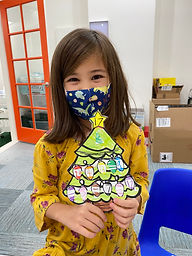## Ms. Brianne

### Target 1​

###### Lesson Type:

New

Number Operation

:

Computation

Solve multi-step addition and subtraction problems within 50.

###### 1:

Solve problems that have more than one operation, addition and subtraction.

###### 2:

Understand when solving a problem that has both addition and subtraction operations that solving starts on the left and continues to the right.

2nd

###### Vocabulary:

Activities:

Students

• solved multi-step addition and subtraction problems
• created their own multi-step addition and subtraction problem as they decorated a Christmas tree, putting the answer in the star at the top of the tree### Home Exploration

###### Guiding Questions:## Absent Students:

### Target 2

:

###### 1:

Study a given arithmetic sequence to determine what rule explains the sequence (i.e., 2-4-6-8-10…each number is the sequence gets bigger by 2).

###### 2:

Write an arithmetic rule to explain a given sequence.

###### 3:

Understand that a pattern can be described by stating the rule that it is following.

3rd

###### Vocabulary:

Sequence, Rule, Pattern

Activities:

Students

• analyzed a given arithmetic sequence to figure out the rule it followed
• used the rule to generate the next numbers in the sequence
• identified the rule using + or -### Home Exploration

###### Guiding Questions:### Target 3

:

###### 1:

Understand that in a coordinate, the first number indicates right and left (column) placement and the second up and down (row) placement.

###### 2:

Identify a row and a column.

###### 3:

Use visual tracking to accurately find the intersection point of a row and column.

3rd

###### Vocabulary:

Coordinates, Row, Column

Activities:

Students

• identified rows and columns in a coordinate grid
• used given coordinates to locate different pictures on the grid### Home Exploration